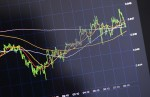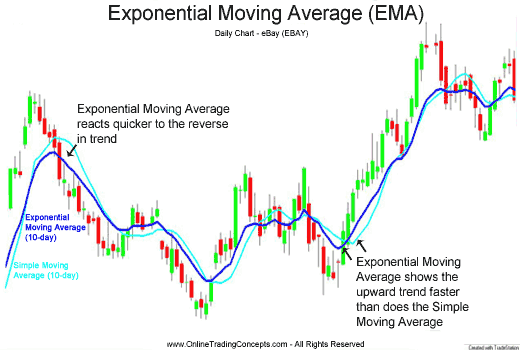# Exponential Moving Average (EMA)Apart from simple and weighted moving average, EMA or exponential moving average is among crucial factors influencing trade in Forex and other financial markets. With moving average in general being the most widely used tool for technical analysis, it is important to discern the different types and their importance, hence the topic at hand.

## What is exponential moving average?

Well, like simple moving average, EMA is calculated using the sum of closing price figures over a number of periods and then divided by the number of said periods. The reason for this similarity is in the title “moving average”, and this is how (moving) average is calculated. However, this is where the “exponential” part comes into play. Unlike simple moving average, exponential moving average shifts the focus toward more recent results, rather than treating them equally. Basically, it means the last few periods are more important than the first few periods (or any periods in between), as the former spearhead the direction of the trend as well as the trend itself. The weight of data shifts as they grow older and new data come in, a process which happens exponentially – hence, the term exponential moving average.## Why is it important?

Before modern computers were developed, simple moving average was the norm when analyzing things such as trends in financial markets. They were easy and straightforward, but they had flaws. Exponential moving average, among others, was a way to correct some of those flaws. Since the main point is to predict the future, rather than statistically describe the past, it stands to reason that recent results should carry more weight than the others. Thus, EMA came into play. It can predict future development with greater certainty than the simple moving average.

## How does it work?

What makes exponential moving average so different from simple moving average is the mathematical formula used to calculate it. Without going into the grueling details, suffice to say each entry gets assigned with a weight index in terms of percentage: the latest entry gets, say, 18%, the previous one gets 15%, and so on while the first one (chronologically) gets, say 3%. The percentage amount never reaches 100% as the data flow is perceived to be indefinite. However, by assigning these percentages to respective entries, we get a result that may differ from the one from simple moving average, which treats all entries equally.

## Conclusion

While there is no objective way to determine which moving average is more effective, or better (“better” being a subjective term in itself), this issue generally comes down to people who prefer simplicity and people who consider new and complex things are superior in the long run. The compromise would be to use all three types of moving averages: simple, weighted and exponential and look for matches: after all, if all three point to the same conclusion, surely it is more reliable than any individual moving average. But then again, it all depends on you.

***Start trading for your chance to win a share of the \$10,000 prize pool. Entering the competition is easy and requires a minimum deposit of only \$100. We will reward the top 6 traders with amazing cash prizes! Register now for your chance to win. Click here!***